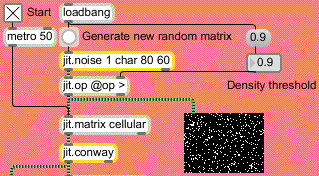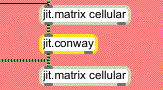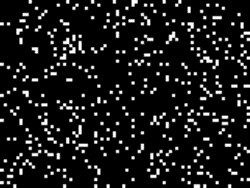Tutorial 17: Feedback Using Named Matrices
This tutorial shows a simple example of using named jit.matrix objects in a feedback loop. We'll use a matrix of random values to seed an iterative process (in this case, Conway's Game of Life).
The tutorial patch generates an initial matrix of randomized values with the jit.noise object:The jit.noise object
The jit.noise object generates a Jitter matrix full of random values. The dim, planecount, and type attributes of the object determine its output matrix (in this instance, we want an 80 x 60 cell matrix of one-plane char data). Our random cell values (which are initially in the range 0 -255) are then set to true (0) or false (255) by the jit.op object. The > operator to jit.op takes the value from the number box (arriving at the right inlet of the object) and uses it as a comparison operator. If a cell value is below that value the cell's value is set to 0. Otherwise the cell is set to 255. Sending a bang to jit.noise will generate a new random matrix.
• Try changing the number box attached to the jit.op object. Click the button attached to the jit.noise object to generate a new matrix each time. Notice how higher comparison values yield fewer white (255) cells. The small jit.pwindow below the jit.op object shows you the random matrix. The one-plane matrix data is correctly interpreted by the jit.pwindow object as grayscale video.
Jitter Matrix Feedback
The quantized noise we've generated at the top of our patch goes from the jit.op object into a jit.matrix object with the name of cellular:Two named jit.matrix objects in a feedback loop
This jit.matrix object, which receives bang messages from a metro object at the top of the patch, is connected to an object called jit.conway, the output of which is hooked up to another jit.matrix with the same name (cellular) as the first. The result of this is that the output of the jit.conway object (whatever it does) is written into the same matrix that its input came from, creating a feedback loop.
• Start the metro object by clicking the toggle box. The jit.pwindow at the bottom of the patch will show you the output of the jit.conway object.
If you want to start with a fresh random matrix, you can always copy a new matrix into the feedback loop by clicking the button attached to thejit.noise object. The matrix from the jit.op object will go into our shared cellular matrix and will be used in the feedback loop.
The Game of Life
The jit.conway object performs a very simple cellular automata algorithm called the 'Game of Life' on an input matrix. Developed by John Conway at Princeton University, the algorithm simulates cycles of organic survival in an environment with a finite food supply. The cells in the matrix are considered either alive (non-0) or dead (0). Each cell is compared with the cells surrounding it in space. If a live cell has two or three live neightbors, it stays alive. If it has more or less than that number, it dies (i.e. is set to 0). If a dead cell has exactly three live neighbors it becomes alive (i.e. is set to 255). It's that simple.
Every time the jit.conway object receives an input matrix it performs one generation of the Game of Life on that matrix. Therefore, it makes sense to use the object inside of a feedback loop, so we can see multiple generations of the algorithm performed on the same initial set of data.
For example, the initial random matrix:Some random matrix values
Generates the following matrices in the first four iterations through the jit.conway object:The first four generations of the Game of Life performed on the dataset above
After seeding the feedback loop with a random matrix, you can turn on the metro object and watch the algorithm run! The Game of Life is designed in such a way that the matrix will eventually stabilize to either a group of self-oscillating cell units or an empty matrix (a dead world). In either case you can just bang in a new set of numbers and start all over again.
Summary
You can use the name attribute of the jit.matrix object to create feedback loops in your Jitter processing. By using two jit.matrix objects with the same name at either end of an object chain, you create a patch where the output of the chain gets written to the same Jitter matrix as the input comes from. The jit.noise object generates matrices of random numbers of any type, dim, or planecount. The jit.conway object, which works best within such a feedback loop, performs simple cellular automatation on an input matrix.